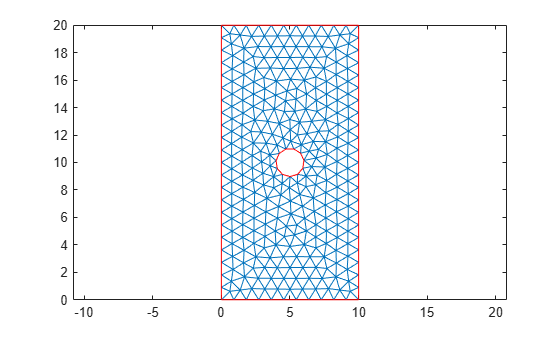# translate

Translate geometry

## Syntax

``translate(g,s)``
``h = translate(g,s)``

## Description

example

````translate(g,s)` translates the geometry `g` by the distance `s`.```
````h = translate(g,s)` returns a handle `h` to the resulting geometry object `g`. If the original geometry is a `DiscreteGeometry` object, then the function modifies the original geometry and returns the handle `h` to the modified `DiscreteGeometry` geometry object. If the original geometry is an `AnalyticGeometry` object, then `h` is a handle to a new `DiscreteGeometry` geometry object. In this case, the original geometry remains unchanged.```

## Examples

collapse all

Translate a geometry by different distances along the x- and y-axes.

Create a model.

`model = createpde;`

Import and plot a geometry.

```g = importGeometry(model,'PlateHolePlanar.stl'); pdegplot(g)```Mesh the geometry and plot the mesh.

```generateMesh(model); figure pdemesh(model)```Translate the 2-D geometry by 1 along the x-axis and by 2 along the y-axis. Plot the result.

```translate(g,[1 2]); pdegplot(g)```Plot the geometry and mesh. The `translate` function modifies a geometry, but it does not modify a mesh.

```figure pdegplot(g) hold on pdemesh(model)```After modifying the geometry, always regenerate the mesh.

```generateMesh(model); figure pdegplot(g) hold on pdemesh(model)```## Input Arguments

collapse all

Geometry, specified as a `DiscreteGeometry` or `AnalyticGeometry` object.

Translation distance, specified as a vector of two or three real numbers. The distance is a vector of two elements for a 2-D geometry or three elements for a 3-D geometry. The elements specify the distance along the x-, y-, and, for a 3-D geometry, z-axes.

## Output Arguments

collapse all

Resulting geometry, returned as a handle. If the original geometry `g` is a `DiscreteGeometry` object, then `h` is a handle to the modified `DiscreteGeometry` geometry object `g`. If `g` is an `AnalyticGeometry` object, then `h` is a handle to a new `DiscreteGeometry` geometry object. In this case, the original geometry `g` remains unchanged.

## Tips

• `translate` modifies a geometry, but it does not modify a mesh. After modifying a geometry, regenerate the mesh to ensure a proper mesh association with the new geometry.

• If `g` is an `AnalyticGeometry` object, and you want to replace it with the resulting discrete geometry, assign the output to the original geometry, for example, `g = translate(g,[1 2])`.